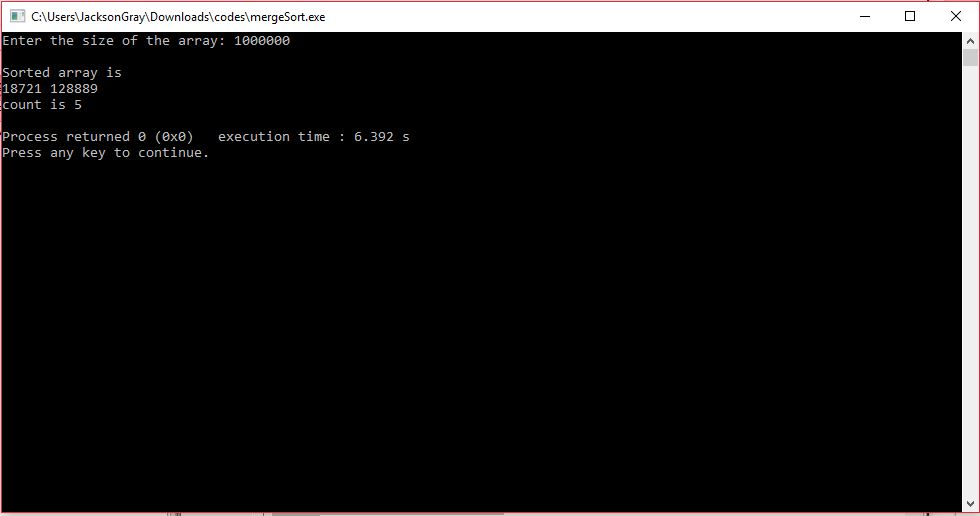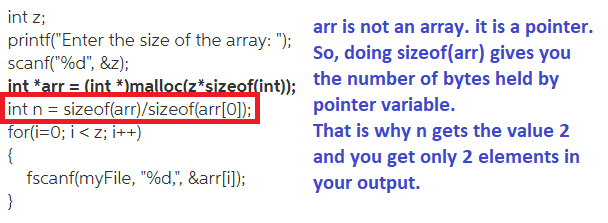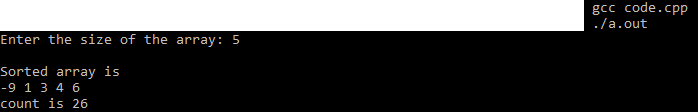# Answered Essay: My c merge code works when I intitalize the array globally at the top of the program, u

My c merge code works when I intitalize the array globally at the top of the program, until the stack overflows. Im trying to initialize the array with malloc, but when i do, the code will only read in 2 integers and stop running. Any help would be appreciated. This program pulls random numbers from a file called alg.txt, and then sorts them. Again, the code works (up until 500k integers) when defining z at the top of the program to the number of integers to be sorted, and declaring the array globally to be equal to arr[z]. Can anyone help me figure out what is going on?

#include<stdlib.h>

#include<stdio.h>

#include<string.h>

int count = 0;

void merge(int arr[], int l, int m, int r)

{

int i, j, k;

int n1 = m – l + 1;

int n2 = r – m;

int L[n1], R[n2];

for (i = 0; i < n1; i++)

L[i] = arr[l + i];

count++;

for (j = 0; j < n2; j++)

R[j] = arr[m + 1+ j];

count++;

i = 0;

j = 0;

k = l;

while (i < n1 && j < n2)

{

if (L[i] <= R[j])

{

arr[k] = L[i];

i++;

count++;

}

else

{

arr[k] = R[j];

j++;

count++;

}

k++;

count++;

}

while (i < n1)

{

arr[k] = L[i];

i++;

k++;

count++;

}

while (j < n2)

{

arr[k] = R[j];

j++;

k++;

count++;

}

}

void mergeSort(int arr[], int l, int r)

{

if (l < r)

{

int m = l+(r-l)/2;

mergeSort(arr, l, m);

mergeSort(arr, m+1, r);

merge(arr, l, m, r);

}

}

void printArray(int A[], int size)

{

int i;

for (i=0; i < size; i++)

printf(“%d “, A[i]);

printf(“n”);

}

int main()

{

int i;

FILE *myFile;

myFile = fopen(“alg.txt”, “r”);

int z;

printf(“Enter the size of the array: “);

scanf(“%d”, &z);

int *arr = (int *)malloc(z*sizeof(int));

int n = sizeof(arr)/sizeof(arr);

for(i=0; i < z; i++)

{

fscanf(myFile, “%d,”, &arr[i]);

}

mergeSort(arr, 0, n – 1);

printf(“nSorted array is n”);

printArray(arr, n);

printf(“count is %dn”, count);

return 0;

}CAUsersVacksonGray Downloadscodes mergeSort.exe Enter the size of the array: 1000000 Sorted array is 18721 128889 count is 5 Process returned e (exe) xcution time 6.392 s Press any key to continue.

The error is that you are using sizeof(arr) where arr is a pointer and not an array.FIX: To fix this, do not calculate n at all. Simply use the value z as the number of elements in the array.

Refer below for the complete working code:

#include<stdlib.h>
#include<stdio.h>
#include<string.h>
int count = 0;
void merge(int arr[], int l, int m, int r)
{
int i, j, k;
int n1 = m – l + 1;
int n2 = r – m;

int L[n1], R[n2];

for (i = 0; i < n1; i++)
L[i] = arr[l + i];
count++;

for (j = 0; j < n2; j++)
R[j] = arr[m + 1+ j];
count++;

i = 0;
j = 0;
k = l;
while (i < n1 && j < n2)
{
if (L[i] <= R[j])
{
arr[k] = L[i];
i++;
count++;
}
else
{
arr[k] = R[j];
j++;
count++;
}
k++;
count++;
}

while (i < n1)
{
arr[k] = L[i];
i++;
k++;
count++;
}

while (j < n2)
{
arr[k] = R[j];
j++;
k++;
count++;
}
}

void mergeSort(int arr[], int l, int r)
{
if (l < r)
{

int m = l+(r-l)/2;

mergeSort(arr, l, m);
mergeSort(arr, m+1, r);

merge(arr, l, m, r);
}
}

void printArray(int A[], int size)
{
int i;
for (i=0; i < size; i++)
printf(“%d “, A[i]);
printf(“n”);
}

int main()
{
int i;
FILE *myFile;
myFile = fopen(“alg.txt”, “r”);

int z;
printf(“Enter the size of the array: “);
scanf(“%d”, &z);
int *arr = (int *)malloc(z*sizeof(int));
int n = sizeof(arr)/sizeof(arr);
for(i=0; i < z; i++)
{
fscanf(myFile, “%d,”, &arr[i]);
}

mergeSort(arr, 0, z – 1);

printf(“nSorted array is n”);
printArray(arr, z);
printf(“count is %dn”, count);
return 0;
}Calculate your paper price
Pages (550 words)
Approximate price: -

Help Me Write My Essay - Reasons:Best Online Essay Writing Service

We strive to give our customers the best online essay writing experience. We Make sure essays are submitted on time and all the instructions are followed.Our Writers are Experienced and Professional

Our essay writing service is founded on professional writers who are on stand by to help you any time.Free Revision Fo all Essays

Sometimes you may require our writers to add on a point to make your essay as customised as possible, we will give you unlimited times to do this. And we will do it for free.Timely Essay(s)

We understand the frustrations that comes with late essays and our writers are extra careful to not violate this term. Our support team is always engauging our writers to help you have your essay ahead of time.Customised Essays &100% Confidential

Our Online writing Service has zero torelance for plagiarised papers. We have plagiarism checking tool that generate plagiarism reports just to make sure you are satisfied.Our agents are ready to help you around the clock. Please feel free to reach out and enquire about anything.

Try it now!

## Calculate the price of your order

Total price:
\$0.00

How it works?

Follow these simple steps to get your paper doneFill in the order form and provide all details of your assignment.Proceed with the payment

Choose the payment system that suits you most.Receive the final file

Once your paper is ready, we will email it to you.

HOW OUR ONLINE ESSAY WRITING SERVICE WORKS

Let us write that nagging essay.## Submit Your Essay/Homework Instructions

By clicking on the "PLACE ORDER" button, tell us your requires. Be precise for an accurate customised essay. You may also upload any reading materials where applicable.Pick A & Writer

Our ordering form will provide you with a list of writers and their feedbacks. At step 2, its time select a writer. Our online agents are on stand by to help you just in case.Editing (OUR PART)

At this stage, our editor will go through your essay and make sure your writer did meet all the instructions.